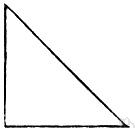# right triangle

Also found in: Thesaurus, Medical, Financial, Acronyms, Encyclopedia, Wikipedia.
Related to right triangle: acute triangle, obtuse triangle

## right triangle

n
(Mathematics) US and Canadian a triangle one angle of which is a right angle. Also called (in Britain and certain other countries): right-angled triangle
Collins English Dictionary – Complete and Unabridged, 12th Edition 2014 © HarperCollins Publishers 1991, 1994, 1998, 2000, 2003, 2006, 2007, 2009, 2011, 2014

## right′ tri′angle

n.
a triangle having a right angle (contrasted with oblique triangle).
[1920–25]

## right triangle

A triangle having a right angle.
ThesaurusAntonymsRelated WordsSynonymsLegend:
 Noun 1right triangle - a triangle with one right angle  right-angled triangletriangle, trigon, trilateral - a three-sided polygonhypotenuse - the side of a right triangle opposite the right angleoblique triangle - a triangle that contains no right angle
Based on WordNet 3.0, Farlex clipart collection. © 2003-2012 Princeton University, Farlex Inc.
Translations
triángulo rectángulo
suorakulmainen kolmio
pravokutni trokut
triangolo rettangolo
dik üçgen
References in periodicals archive ?
Caption: FIG 3: Schematic Illustration of Viscoelastic Properties: The complex modulus (or Young's modulus) can be thought of as the hypotenuse of a right triangle, while the elastic and viscous modulus represent the other two sides.
Chapter 2 addresses the question whether Thales plausibly knew of "an areal interpretation" of the Pythagorean theorem, that is, an interpretation that regards the relations between the sides of a right triangle in terms of areas subtended by its sides.
The nominal height of the microstructure is 25 [micro]m, the period is 50 [micro]m, and the shape is equilateral right triangle.
(d) The generic right triangle case: we select O = (0, 0), B = (3, 0), and A = (3, 5), as shown in Figure 4(d).
Adding two Pythagorean triples: Let A: (x, y, r) and B: (X, Y, R) be two Pythagorean triples (right triangle sides that are integers) shown below in the two triangles.
Check out its "20 GIFs of How Things Work,'' particularly the water container proof of Pythagoras' formula for a right triangle. Also the "Stunning Staircases in Abandoned Mansions.''
Similarly, several, if not all, English letters are, simply by tradition or abbreviation, commonly associated with mathematical formulae, and thus, if one wished to express, in a phrase, the letter c, one could write [square root]([a.sup.2] + [b.sup.2]), relying on the reader's knowledge of the Pythagorean Theorem to connect the meaningless letters with the known equation "[a.sup.2] + [b.sup.2] = [c.sup.2]," even though the letters themselves may stand for anything at all and bear no necessary relation to the sides of a right triangle with no context.
For a right triangle with base of 20 and height of one, the angle is arctan(1/20) or 2.86 degrees.
Left (east) of the Sickle (by more than a fist-width at arm's length) is Leo's back end, three bright stars m a right triangle.
Let's consider a right triangle with one of its legs along the motion direction (Fig.
Euclid's theorem of leg: For every right triangle square of the length of the leg is equal to the product of the hypotenuse and the segment of the hypotenuse adjacent to the leg.

Site: Follow: Share:
Open / Close